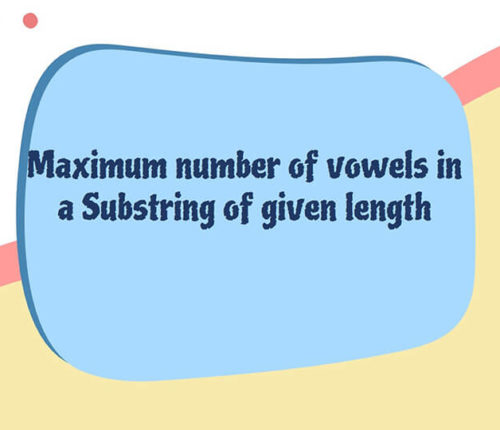# Count 1’s in sorted Binary Array

If you want to practice data structure and algorithm programs, you can go through Java coding interview questions.

In this post, we will see how to count number of 1’s in sorted binary array.

## Problem

Print number of 1’s in a given sorted Binary Array.
For eg:-

int[] arr = {0,0,0,1,1,1,1};
output : 4

int[] arr = {0,0,1};
output : 1

## Solution

The Naive solution to solve this problem would be looping in the array and keep a count of 1’s occurring in the array.
But as the array is sorted we can stop at the very first one encountered, because as the array is sorted we can be sure that the element after first one would all be greater than or equal to ones, but as our array contains zeroes and ones only, therefore all elements after first one would be one. So our answer would be (array.length – currentPointer). This will handle all the test cases and works in linear O(n) time.

We can still solve the problem more efficiently in logarithmic time i.e. with a worst time complexity of O(log n).

Efficient Approach :

We can use divide and conquer approach, and move recursively at every step dividing the array into two subarrays virtually by keeping our start and end pointers.

1.  If the element at end pointer of a subarray is zero this means that all the elements in that array would be zeroes and we know the array is already sorted.
2. What if the first element that is the value at start pointer of the array? this means that all the elements in that subarray are ones again keeping in mind that array is sorted.

Now let’s discuss the time complexity.
At every call we are basically dividing the number of elements to work upon into two halves.
Initially we had N elements, then N/2, then N/4 and so on until we have one element left to work with.

if we denote T(n) for the time taken to process n elements.
Mathematically, we can write the equations as:

T(n) = T(n/2) + T(n/2)
T(n/2) = T(n/4) + T(n/4)
.
.
.
T(2) = T(1) + T(1)

Say we have x such equations and as we move up in equations, number of elements are getting doubled, so eventually,
n = 2^x
taking log on both sides,
x = log(n) {to the base 2}
Hence the complexity of our algorithm comes out to be O(log(n)).

When you run above program, you will get below output

7 0 0 0 1 1 1 1
arr[]: { 0 0 0 1 1 1 1 }
Number of 1 in array :4

That’s all about how to Count 1’s in sorted Binary Array
Comment in case of any doubt or edit. Happy Learning 🙂

import_contacts

### You may also like:## Related Posts

•18 June

### Maximum Number of Vowels in a Substring of Given Length

Table of ContentsApproach – 1 Generate All Substrings Using substring() MethodApproach – 2 Using Sliding Window Method (Linear Time Solution) In this article, we will look at an interesting problem related to the Strings and [Sliding-Window Algorithm](https://java2blog.com/sliding-window-maximum-java/ “Sliding-Window Algorithm”). The problem is : "Given a String we have to Find the Maximum Number of Vowel […]

•04 June

### Search for a range Leetcode – Find first and last position of element in sorted array

Table of ContentsApproach 1 (Using Linear Search)Approach 2 (Using Modified Binary Search-Optimal) In this article, we will look into an interesting problem asked in Coding Interviews related to Searching Algorithms. The problem is: Given a Sorted Array, we need to find the first and last position of an element in Sorted array. This problem is […]

•30 April

### Convert Postfix to Infix in Java

Learn about how to convert Postfix to Infix in java.

•30 April

### Convert Prefix to Postfix in Java

Learn about how to convert Prefix to Postfix in java.

•16 April

### Data Structures in java

•